# Orient noise pattern along vectors

## Recommended Posts

I aligned vector directions towards some curves (ie. outer edges). How would I orient a stretched noise pattern accordingly?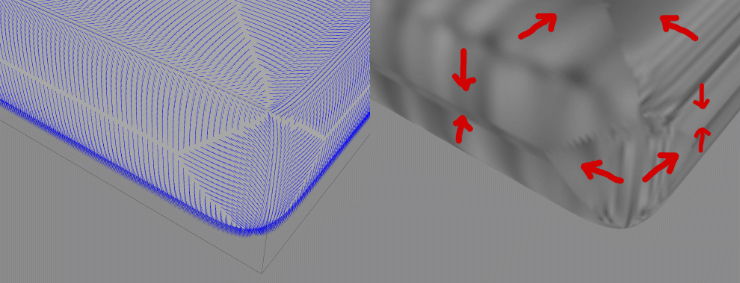oriented_noise.hiplc

##### Share on other sites

• 3 weeks later...

I have made some progress in making the wrinkles run towards the outer edges. Unfortunately i still get lots of warping issues that I can't quite explain.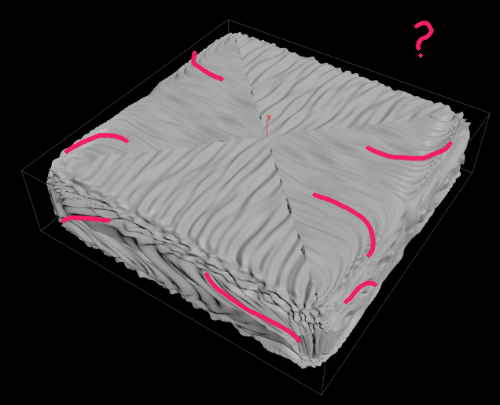Ideally I want all folds to run in a parallel manner towards their nearest outer edge. This is the code I am currently using:

```vector pos_b = minpos(1, v@P);

vector dir = normalize(pos_b - v@P);
vector rect = cross(v@N, dir);
vector nml = cross(dir, rect);
matrix3 rot = set(dir, nml, rect);

vector freq = {8, 1, 40};
vector pos = rot * v@P * freq;

float deform = noise(pos);
v@P += v@N * deform * 0.05;```
Edited by konstantin magnus
•1
##### Share on other sites

You should normalize your crossed vectors as they are not unit size.

Also, order of your operations matters. If you want to 'replicate' your tree on the left, then probably you would like to:

```vector pos_b = minpos(1, v@P);

vector dir = rint(normalize(pos_b - v@P));
vector rect = normalize(cross(v@N, dir));
vector nml = normalize(cross(dir, rect));
matrix3 rot = set(dir, nml, rect);

vector freq = {8,1, 40};
vector pos = (rot * v@P) * freq;

float deform = noise(pos);
v@P += v@N * deform * 0.05;```

Although that probably still would not solve your initial issue

Edited by tmdag
##### Share on other sites

Thanks for your help @tmdag. I had to invert the rotation matrix. So its:

```vector freq  = chv('frequency');

vector dir   = normalize( minpos(1, v@P) - v@P );
vector rect  = normalize( cross(v@N, dir) );
vector nml   = normalize( cross(dir, rect) );
matrix3 rot  = set(dir, nml, rect);
float deform = noise(v@P * invert(rot) * freq);

v@P += v@N * deform * 0.05;```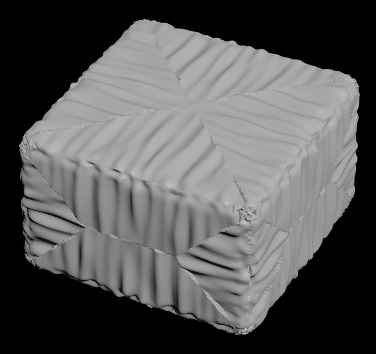Edited by konstantin magnus
•1
##### Share on other sites

Hi. How about computing local space per primitive instead, and then get noise position from point position in the local space? Some sort of edge based UV unwrap.

```// Primitive wrangle.

int pts[] = primpoints(0, @primnum);

// Compute averaged primitive normal from point normals computed from their neighbours.
vector normals[];
foreach (int pt; pts)
{
vector normalized_edges[];
vector pt_pos = point(0, "P", pt);
foreach (int nb; neighbours(0, pt))
{
vector nb_pos = point(0, "P", nb);
append(normalized_edges, normalize(pt_pos - nb_pos));
}
append(normals, normalize(avg(normalized_edges)));
}
vector normal = normalize(avg(normals));

// Compute edge tangent.
vector pt0 = point(0, "P", pts);
vector pt1 = point(0, "P", pts);
vector edge = normalize(pt0 - pt1);

// Compute bitangent and orthonormalize matrix.
vector perp = normalize(cross(normal, edge));
normal = normalize(cross(edge, perp));

3@tangent_space = set(perp, normal, edge);```

Final deformation code:

```// Point wrangle.

int prim;
xyzdist(1, @P, prim, set(0));
matrix3 tangent_space = prim(1, "tangent_space", prim);

vector pos = @P * invert(tangent_space);
float deform = noise(pos * {10,1,100}) * 0.05;
v@P += v@N * deform;```

Some image sampling could work too:

tangent_space_noise.hipnc

Edited by f1480187
•4
•4
##### Share on other sites

Great idea @f1480187, creating the rotation matrices from the cage lines is definitely cleaner!

##### Share on other sites

• 1 year later...

I tried using guiding my hard surface looking VOP noise or COP input using custom curves and this approach and @f1480187 awesome VEX goodie, but I couldn t get a satisfying result.

- Basically, my intention is to orient/distort my noise to follow my geometry flow. So i could extract curve from gradient ascent/descent and curvature and use these.

* I like to use some field like curvature / gradient to sculpt my geo so well obviously i don't need UV  and more importantly i could sure the details added in in relation with the form, "flow "with the mesh...   Interesting, i could get something out of that now the lines could be hard to control, messy.

* So second way to process will be to use noise input, via substance, COP,s noise VOP or textures then guide this noise to flow around correctly. Ok, usually the way i know is to define seams and work on the UV to be as straight, vertical or horizontal as possible, less distorsion as possible obviously. Now well to make sure the noise is going to join in an acceptable way along the seam is more difficult without hand painting to my knowledge.

Do some of you have face the same issues and questions ?My initial idea was to advect using a velocity field from the gradient or/ and curvature##### Share on other sites

Also quite related i believe, here s an interesting approach to compute weathering @konstantin magnus

"the pointiness of the whole mesh is computed and normalizes it from the most convex to the most concave area of the mesh. It gives 6 different 2dimensional UVMaps, giving direction along the Surface. And it gives smooth gradients for dirtflow even if the surface is flat, leading to more complex results. In the right image a displaced housewall is mapped with gradients using the "segments", "dirt" and "flow-gather" map. Though it is mainly flat areas, the add-on generates smooth gradients along them so the dirt flow naturally along" the pointiness of the whole mesh and normalizes it from the most convex to the most concave area of the mesh. It gives 6 different 2dimensional UVMaps, giving direction along the Surface. And it gives smooth gradients for dirtflow even if the surface is flat, leading to more complex results. In the right image a displaced housewall is mapped with gradients using the "segments", "dirt" and "flow-gather" map. Though it is mainly flat areas, the add-on generates smooth gradients along them.

Im not quite sure what are and how to compute what are the gathermap and segmentmap. For the flowmap, is it computed from the dirt map and with a vector field do you think? Excepted simulation, what will be your take on this guys if i could ask your opinion? Cheers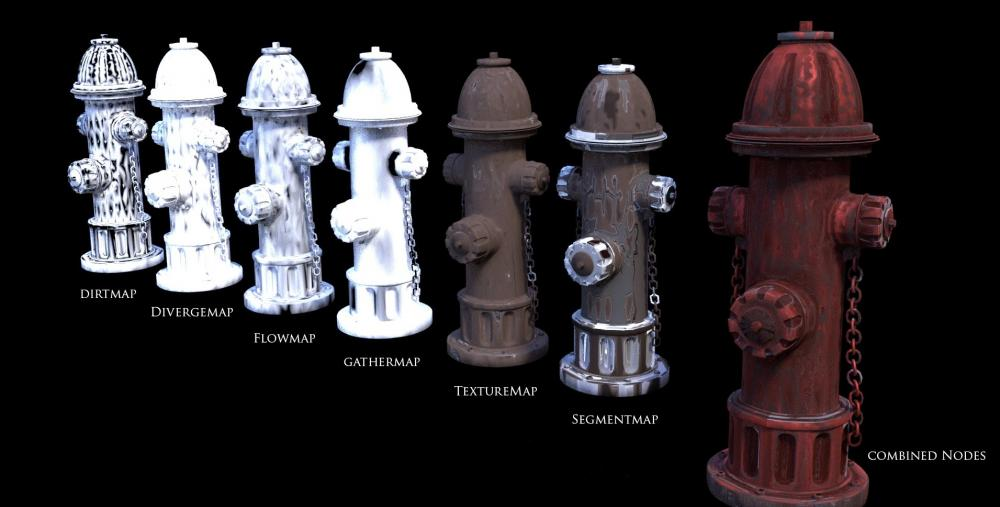•1
##### Share on other sites

I think i will tonight, try to compute the luma gradient of the curvature (with noise breakup) or/and occlusion(dirt map) and advect it , maybe deform by a curl.

I don't think i will get something like the map i was asking questions but maybe something interesting from that... ?

Other suggestion are welcomed

Vincent Thomas   (VFX and Art since 1998)

Edited by vinyvince
##### Share on other sites

Potential flow * curvature -> Color Advection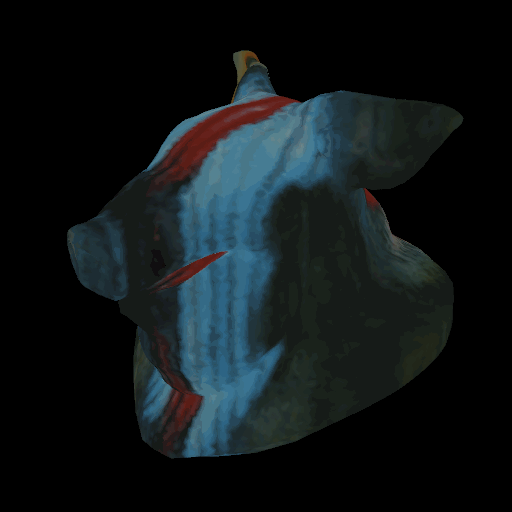This has changed from the original agenda, so I think you need to start a new thread.

Edited by satoru
##### Share on other sites

On 09/10/2020 at 5:21 AM, satoru said:

Potential flow * curvature -> Color Advection

This has changed from the original agenda, so I think you need to start a new thread.

Yep, what i have in mind and tried to explained earlierI think it looks natural and a good start for a weathering effect @satoru

Edited by vinyvince

## Join the conversation

You can post now and register later. If you have an account, sign in now to post with your account.
Note: Your post will require moderator approval before it will be visible.×   Pasted as rich text.   Paste as plain text instead

Only 75 emoji are allowed.

×   Your previous content has been restored.   Clear editor

×   You cannot paste images directly. Upload or insert images from URL.

×
×